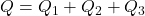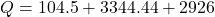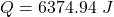## The following physical constants are for water, H2O. The specific heat capacity of the solid = 2.09 J/g oC The specific heat ca

Question

The following physical constants are for water, H2O.
The specific heat capacity of the solid = 2.09 J/g oC
The specific heat capacity of the liquid = 4.18 J/g oC
The specific heat capacity of the vapor = 2.09 J/g oC
∆Hfus = 6.02 kJ/mol; ∆Hvap = 40.7 kJ/mol Freezing point = 0.0oC; Boiling point = 100.0oC
How much heat(in kJ) is required to warm 10.0 grams of ice at -5.0oC to a temperature of 70.0oC?

in progress 0
6 months 2021-07-13T19:26:19+00:00 1 Answers 0 views 0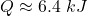Explanation:

Quantity of heat required by 10 gram of ice initially warm it from -5°C to 0°C: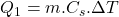here;

mass, m = 10 g

specific heat capacity of ice,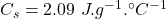change in temperature,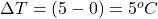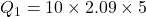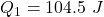Amount of heat required to melt the ice at 0°C: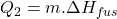where,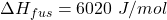we know that no. of moles is = (wt. in gram)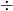(molecular mass)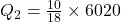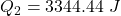Now, the heat required to bring the water to 70°C from 0°C: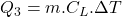specific heat of water,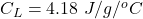change in temperature,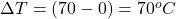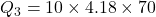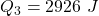Therefore the total heat required to warm 10.0 grams of ice at -5.0°C to a temperature of 70.0°C: